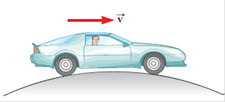Suppose a 1 800-kg car passes over a bump in a roadway that follows the arc of a circle of radius 20.4 m, as in Figure P7.65. (a) What force does the road exert on the car as the car passes the highest point of the bump if the car travels at 8.94 m/s? (b) What is the maximum speed the car can have without losing contact with the road as it passes this highest point?FIGURE P7.65

Question

Suppose a 1 800-kg car passes over a bump in a roadway that follows the arc of a circle of radius 20.4 m, as in Figure P7.65. (a) What force does the road exert on the car as the car passes the highest point of the bump if the car travels at 8.94 m/s? (b) What is the maximum speed the car can have without losing contact with the road as it passes this highest point?

FIGURE P7.65